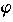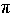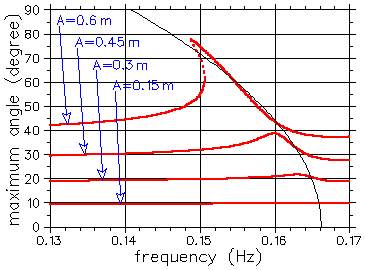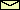Nonlinear Resonance

At the first glance, nonlinear resonance seems not to be so much different from the (linear) resonance of a harmonic oscillator. But both, the dependency of the eigenfrequency of a nonlinear oscillator on the amplitude and the nonharmoniticity of the oscillation lead to a behavior that is impossible in harmonic oscillators, namely, the foldover effect and superharmonic resonance, respectively. Both effects are especially important in the case of weak damping.

1. The foldover effect

 g = 9.81 m/sec2,  l = 1 m,= 0.4 sec-1The foldover effect got its name from the bending of the resonance peak in a amplitude versus frequency plot. This bending is due to the frequency-amplitude relation which is typical for nonlinear oscillators. As an example, the figure on the left shows a sinusoidally driven pendulum. As long as the driving amplitude A is small, the resonance line is very well approximated by the result for an underdamped harmonic oscillator. For a stronger driving, the resonance lines "folds", leading to bistability and hysteresis. That is, the nonlinear oscillator oscillates either with a large amplitude or a small amplitude. Inbetween there is always an unstable periodic solution (shown as a dotted line). At the ends of the interval of bistablity this unstable limit cycle annihilates with one of its stable counterpart in a saddle-node bifurcation. The foldover effect also occurs in superharmonic resonance and parametric resonance.

The nonlinear resonance line can be approximated considerably well (see the green line in the figure) by the following heuristic approach: Take the resonance line of the harmonic oscillator and replace the eigenfrequency0 by the frequency-amplitude relationres(max) = 2/T (shown as a thin black line in the figure). This approximation assumes that the oscillation is still harmonic, i.e., sinusoidal, but the frequency depends on the amplitude. The figure shows that this approximation works quantitatively well even for large values ofmax. By the way, the most easiest way to plot this approximation is to draw f as a function ofmax because the equation for the resonance line is a quadratic in f 2.

For small values of the damping constant, the onset Ac of bistability is proportional to some power of. A power-law in the frequency-amplitude relation, i.e.,

(res -0)  ~  (max) s

Ac  ~1+1/s ,

because the width and the height of the resonance line are proportional toand A/, respectively.

2. Superharmonic resonance

 g = 9.81 m/sec2,  l = 1 m,= 0.1 sec-1Nonlinear oscillators do not oscillate sinusoidal. Their oscillation is a sum of harmonic (i.e., sinusoidal) oscillations with frequencies which are integer multiples of the fundamental frequency (i.e., the inverse of the period of the nonlinear oscillation). This is the well-known theorem of Jean Baptiste Joseph Fourier (1768-1830) which says that periodic functions can be written as (infinite) sums (so-called Fourier series) of sinus and cosinus functions. Superharmonic resonance is simply the resonance with one of this higher harmonics of a nonlinear oscillation. In a plot of oscillation amplitude versus driving frequency, you can, therefore, expect additional resonance peaks. In general, they appear at driving frequencies which are integer fractions of the fundamental frequency.

If you try to find them for the pendulum driven by a periodic force, you have to choose relatively large driving amplitudes and low values for the damping constant. Nevertheless, you will not find superharmonic resonance at half the eigenfrequency of the pendulum! Why? The reason is symmetry. The undriven pendulum is invariant under reflection symmetry. That is, if you change the sign of, the equation of motion remains unchanged. Due to this symmetry, the nonlinear oscillation of the pendulum does not contain frequencies which are even multiples of the fundamental frequency. Thus, the first superharmonic resonance occurs near one third of the fundamental frequency. Again, the foldover effect occurs.

 QUESTIONS worth to think about: What happens in a nonlinear oscillator driven nonlinearly as for pendula driven by periodic motions of their suspension point? Will the foldover effect and superharmonic resonance also occur in these cases or not? Will there be additional resonance lines as for the case of a pendulum driven by a sinusoidal force?

© 1998 Franz-Josef Elmer,Franz-Josef doht Elmer aht unibas doht ch, last modified Monday, July 20, 1998.